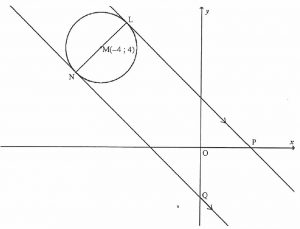The line LP with equation   y + x – 2 = 0 is a tangent at L to the circle with centre M(-4 , 4).

LN is a diameter of the circle.

LP is parallel to NQ where P is on the x-axis and Q is on the y-axis.

What equations and coordinates can you find?

The length of the diameter is doubled and the circle is translated 6 units to the right. Find the equation of the new circle.

Have you discovered all that is possible? Click here to find out.

Tagged with: